### Infinite body with a spherical void, steady 1-D.

RS10 Infinite body surrounding a spherical void, a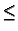r <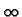, with G = 0 (Dirichlet) at r = a.

4GRS10(r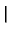r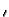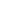) =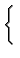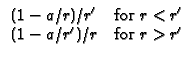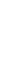RS20 Infinite body surrounding a spherical void, ar <, with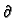G/r = 0 (Neumann) at r = a.

4GRS20(rr) =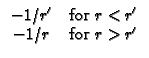RS30 Infinite body surrounding a spherical void, `a < r <, with - kG/r + h1G = 0 (convection) at r = a. Note B1 = h1a/k.

4GRS30(rr) =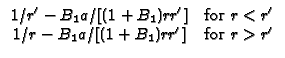Kevin D. Cole
2002-12-31